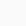# MATLAB for Beginners A Gentle Approach Revised Edition

PDF Free Download | MATLAB for Beginners A Gentle Approach Revised Edition by Pete I. Kattan

## Preface to MATLAB for Beginners eBook

This book is written for people who wish to learn MATLAB 1 for the first time. The book is really designed for beginners and students.

In addition, the book is suitable for students and researchers in various disciplines ranging from engineers and scientists to biologists and environmental scientists.

The book is intended to be used as a first course in MATLAB in these areas.

Students at both the undergraduate level and graduate level may benefit from this book.

The material presented has been simplified to such a degree such that the book may also be used for students and teachers in high schools – at least for performing simple arithmetic and algebraic manipulations.

One of the objectives of writing this book is to introduce MATLAB and its powerful and simple computational abilities to students in high schools.

The material presented increases gradually in difficulty from the first few chapters on simple arithmetic operations to the last two chapters on solving equations and an introduction to calculus.

In particular, the material presented in this book culminates in Chapters 6 and 7 on vectors and matrices, respectively. There is no discussion of strings, character variables or logical operators in this book.

The emphasis is on computational and symbolic aspects. In addition, the MATLAB Symbolic Math Toolbox 2 is emphasized in this book.

A section is added at the end of each chapter (except chapters 1 and 9) dealing with various aspects of algebraic and symbolic computations with the Symbolic Math Toolbox.

In fact, the final chapter on calculus assumes entirely the use of this toolbox.

Chapter 1 provides an overview of MATLAB and may be skipped upon a first reading of the book. The actual material in sequence starts in Chapter 2 which deals with arithmetic operations.

Variables are introduced in Chapter 3 followed by mathematical functions in Chapter 4. The important topic of complex numbers is covered in Chapter 5.

This is followed by Chapters 6 and 7 on vectors and matrices, respectively, which provide the main core of the book. In fact, MATLAB stands for MATrix LABoratory – thus a long chapter is devoted to matrices and matrix computations.

An introduction to programming using MATLAB is provided in Chapter 8. Plotting two-dimensional and three-dimensional graphs is covered in some detail in Chapter 9.

Finally, the last two chapters (10 and 11) present solving equations and an introduction to calculus using MATLAB.

The material presented in this book has been tested with version 7 of MATLAB and should work with any prior or later versions.

There are also over 230 exercises at the ends of chapters for students to practice. Detailed solutions to all the exercises are provided in the second half of the book.

The material presented is very easy and simple to understand – written in a gentle manner.

An extensive references list is also provided at the end of the book with references to books and numerous web links for more information.

This book will definitely get you started in MATLAB. The references provided will guide you to other resources where you can get more information.

Share book:

## Related Free Books## Book Details

Author

Language

Pages

Size

Format

CategoryWhat's the problem with this file?# Attention !

## Help us remove any copyrighted material that may be posted on our site.

We ask you to inform us of any infringing material that may be unintentionally published on our site to remove it.

Reach us at: [email protected]

# Attention !

## Help us remove any copyrighted material that may be posted on our site.

We ask you to inform us of any infringing material that may be unintentionally published on our site to remove it.

Reach us at: [email protected]